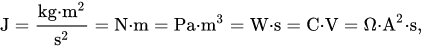# Joule (J) unit

### Joule definition

The joule is a derived unit of energy in the International System of Units. It is equal to the energy transferred to (or work done on) an object when a force of one newton acts on that object in the direction of the force's motion through a distance of one metre (1 newton metre or N⋅m). It is also the energy dissipated as heat when an electric current of one ampere passes through a resistance of one ohm for one second. It is named after the English physicist James Prescott Joule (1818–1889).One joule can also be defined as the following:

• The work required to move an electric charge of one coulomb through an electrical potential difference of one volt, or one coulomb-volt (C⋅V). This relationship can be used to define the volt.
• The work required to produce one watt of power for one second, or one watt-second (W⋅s) (compare kilowatt-hour – 3.6 megajoules). This relationship can be used to define the watt.

The joule is named after James Prescott Joule. As with every SI unit named for a person, its symbol starts with an upper case letter (J), but when written in full it follows the rules for capitalisation of a common noun; i.e., "joule" becomes capitalised at the beginning of a sentence and in titles, but is otherwise in lower case.

### Practical examples

One joule represents (approximately)

• The kinetic energy of a 2 kg mass traveling at 1 m/s
• The energy required to lift a medium-sized tomato up 1 metre (3 ft 3 in) (assume the tomato has a mass of approximately 100 grams (3.5 oz)).
• The energy required to accelerate a 1 kg mass at 1 m⋅s−2 through a distance of 1 m.
• The heat required to raise the temperature of 1 g of water by 0.24 °C.
• The typical energy released as heat by a person at rest every 1/60 s (approximately 17 ms).
• The kinetic energy of a 50 kg human moving very slowly (0.2 m/s or 0.72 km/h).
• The kinetic energy of a 56 g tennis ball moving at 6 m/s (22 km/h).
• The amount of electricity required to light a 1 W LED for 1 s.

A kilowatt-hour is 3.6 megajoules.

### Multiples of joule

source: https://en.wikipedia.org/wiki/Joule

Submultiples Multiples Value SI symbol Name Value 10−1 J dJ decijoule 101 J daJ decajoule 10−2 J cJ centijoule 102 J hJ hectojoule 10−3 J mJ millijoule 103 J kJ kilojoule 10−6 J µJ microjoule 106 J MJ megajoule 10−9 J nJ nanojoule 109 J GJ gigajoule 10−12 J pJ picojoule 1012 J TJ terajoule 10−15 J fJ femtojoule 1015 J PJ petajoule 10−18 J aJ attojoule 1018 J EJ exajoule 10−21 J zJ zeptojoule 1021 J ZJ zettajoule 10−24 J yJ yoctojoule 1024 J YJ yottajoule Common multiples are in bold face

### Conversions

107 erg (exactly)

6.24150974×1018 eV

0.2390 cal (gram calories)

2.390×10-4 kcal (food calories)

9.4782×10-4 BTU

0.7376 ft⋅lb (foot-pound)

23.7 ft⋅pdl (foot-poundal)

2.7778×10-7 kW⋅h (kilowatt-hour)

2.7778×10−4 W⋅h (watt-hour)

9.8692×10−3  l⋅atm (litre-atmosphere)

11.1265×10−15  g (by way of mass-energy equivalence)

10−44  foe (exactly)

Currently, we have around 5612 calculators, conversion tables and usefull online tools and software features for students, teaching and teachers, designers and simply for everyone.